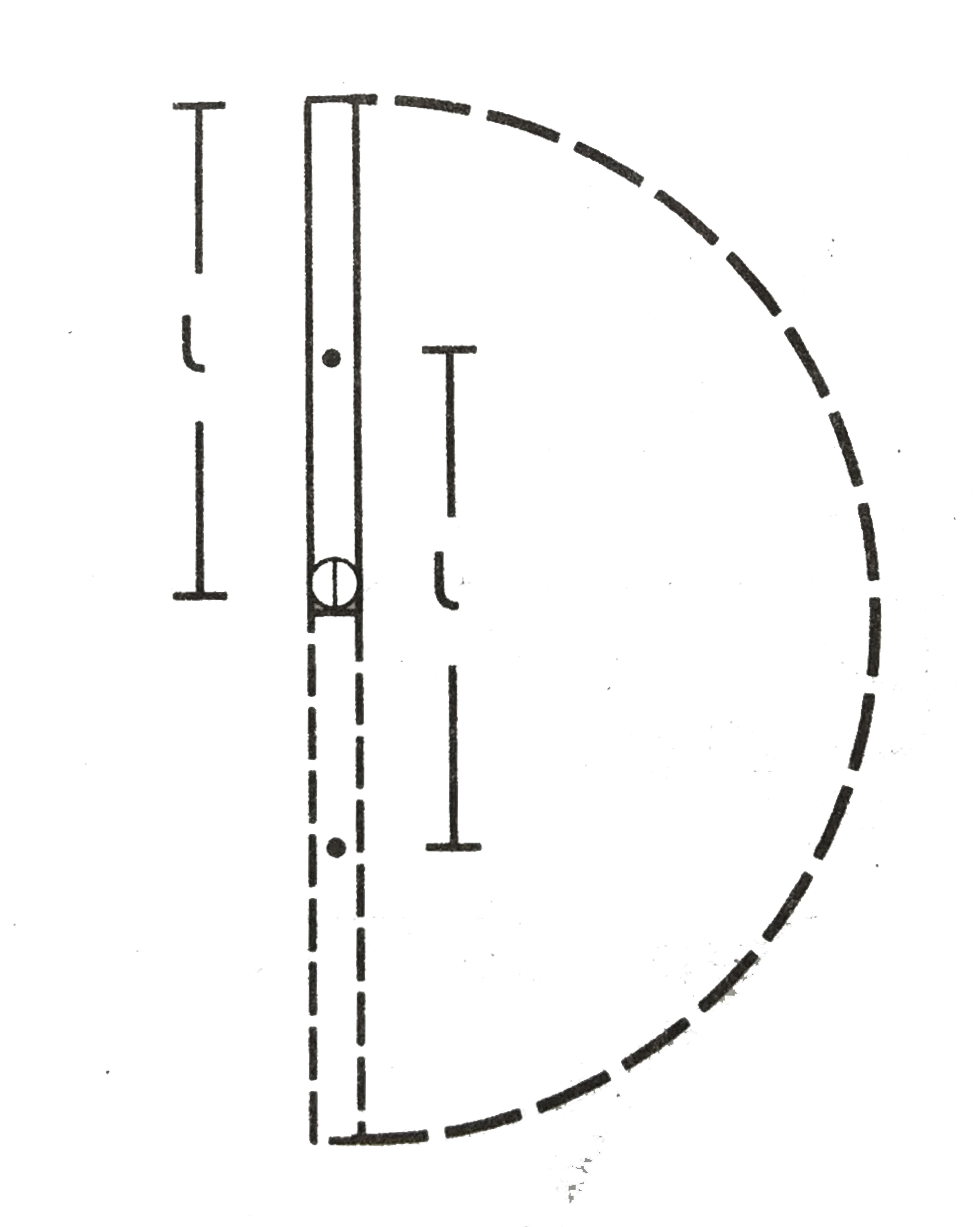# A uniform rod of mas m and length l is kept vertical with the lower end clamed. It is slightly pushed to let it fall down under gravity. Find its angu

40 views
in Physics
closed
A uniform rod of mas m and length l is kept vertical with the lower end clamed. It is slightly pushed to let it fall down under gravity. Find its angular speed when the rod is passing through its lowest positon. Neglect any friction at the clamp. What will be the linear speed of the free end at this instant?

by (75.0k points)
selectedAs the rod reaches its lowest position, the centre of mass is lowered by a distance l. Its gravitatioN/Al potential energy is decreased by mgl. As no energy lost against friction, this should be equal to the increase in the kinetic energy. As the rotation ocfurs about the horiztonsal axis through the clamped end, the moment of inertia is I=ml^2/3. Thus
1/2Iomega^2=mgl
1/2((ml^2)/3)omega^2=mgl
or, omega sqrt(6g)/l
the linear speed of the free end is
v=lometa=sqrt(6gl)